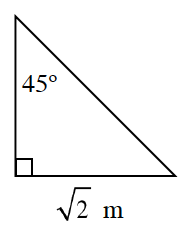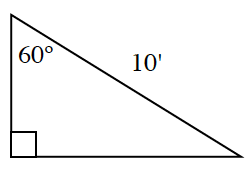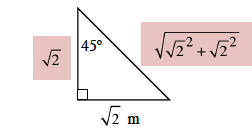### Home > CCG > Chapter 5 > Lesson 5.2.1 > Problem5-52

5-52.

For each triangle below, use your triangle shortcuts from this lesson to find the missing side lengths. Then find the area and perimeter of the triangle.

1.1.What special triangles can you use for each part?
Find the lengths of the missing sides using the special triangle relationship you chose.

Perimeter Calculation$\text{Perimeter }= 2 + \sqrt{2} + \sqrt{2}$

$2 + 2\sqrt2$ m

Area Calculation

$\text{Area }=\frac{1}{2}\cdot b\cdot h$

$=\frac{1}{2}\sqrt{2} \cdot\sqrt{2}$

$=\frac{1}{2}\cdot (2)$

$\text{Area}=1$ sq. m